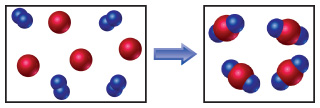# Problem: The reaction between reactant A (blue spheres) and reactant B (red spheres) is shown in the following diagram:Based on this diagram, which equation best describes the reaction?(a) A2 + B → A2B(b) A2 + 4B → 2AB2(c) 2A + B4 → 2AB2(d) A + B2 → AB2

###### FREE Expert Solution

Reactant

4 B

4 A2

Product

4 A2B

95% (95 ratings)###### Problem Details

The reaction between reactant A (blue spheres) and reactant B (red spheres) is shown in the following diagram:Based on this diagram, which equation best describes the reaction?

(a) A2 + B → A2B

(b) A2 + 4B → 2AB2

(c) 2A + B4 → 2AB2

(d) A + B2 → AB2

Frequently Asked Questions

What scientific concept do you need to know in order to solve this problem?

Our tutors have indicated that to solve this problem you will need to apply the Balancing Chemical Equations concept. You can view video lessons to learn Balancing Chemical Equations. Or if you need more Balancing Chemical Equations practice, you can also practice Balancing Chemical Equations practice problems.

What professor is this problem relevant for?

Based on our data, we think this problem is relevant for Professor Gulde's class at UB.